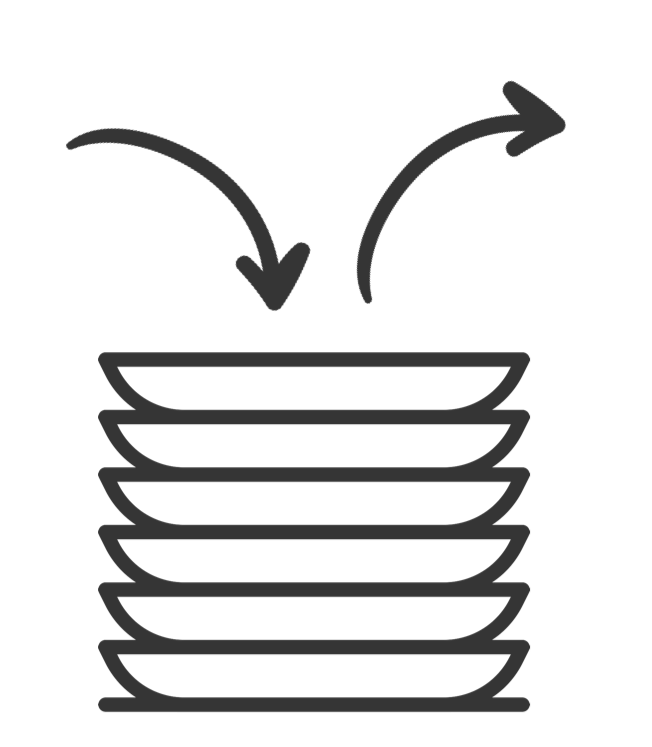# Let's Build a Stack¶

In this lab we will build a stack data structure and use the SPARK provers to find the errors in the below implementation.

## Background¶

So, what is a stack?

A stack is like a pile of dishes...1. The pile starts out empty.
2. You add ( push ) a new plate ( data ) to the stack by placing it on the top of the pile.
3. To get plates ( data ) out, you take the one off the top of the pile ( pop ).
4. Out stack has a maximum height ( size ) of 9 dishes

Pushing items onto the stack

Here's what should happen if we pushed the string MLH onto the stack.

The list starts out empty. Each time we push a character onto the stack, Last increments by 1.

Popping items from the stack

Here's what should happen if we popped 2 characters off our stack & then clear it.

Note that pop and clear don't unset the Storage array's elements, they just change the value of Last.

## Input Format¶

N inputs will be read from stdin/console as inputs, C to the stack.

## Constraints¶

1 <= N <= 1000 C is any character. Characters d and p will be special characters corresponding to the below commands:

p => Pops a character off the stack

d => Prints the current characters in the stack

## Output Format¶

If the stack currently has the characters "M", "L", and "H" then the program should print the stack like this:

[M, L, H]

## Sample Input¶

M L H d p d p d p d

## Sample Output¶

[M, L, H] [M, L] [M] []

package Stack with SPARK_Mode => On is procedure Push (V : Character) with Pre => not Full, Post => Size = Size'Old + 1; procedure Pop (V : out Character) with Pre => not Empty, Post => Size = Size'Old - 1; procedure Clear with Post => Size = 0; function Top return Character with Post => Top'Result = Tab(Last); Max_Size : constant := 9; -- The stack size. Last : Integer range 0 .. Max_Size := 0; -- Indicates the top of the stack. When 0 the stack is empty. Tab : array (1 .. Max_Size) of Character; -- The stack. We push and pop pointers to Values. function Full return Boolean is (Last = Max_Size); function Empty return Boolean is (Last < 1); function Size return Integer is (Last); end Stack;
package body Stack with SPARK_Mode => On is ----------- -- Clear -- ----------- procedure Clear is begin Last := Tab'First; end Clear; ---------- -- Push -- ---------- procedure Push (V : Character) is begin Tab (Last) := V; end Push; --------- -- Pop -- --------- procedure Pop (V : out Character) is begin Last := Last - 1; V := Tab (Last); end Pop; --------- -- Top -- --------- function Top return Character is begin return Tab (1); end Top; end Stack;
with Ada.Command_Line; use Ada.Command_Line; with Ada.Text_IO; use Ada.Text_IO; with Stack; use Stack; procedure Main with SPARK_Mode => Off is ----------- -- Debug -- ----------- procedure Debug is begin if not Stack.Empty then Put ("["); for I in Stack.Tab'First .. Stack.Size - 1 loop Put (Stack.Tab (I) & ", "); end loop; Put_Line (Stack.Tab (Stack.Size) & "]"); else Put_Line ("[]"); end if; end Debug; S : Character; begin ---------- -- Main -- ---------- for Arg in 1 .. Argument_Count loop if Argument (Arg)'Length /= 1 then Put_Line (Argument (Arg) & " is an invalid input to the stack."); else S := Argument (Arg)(Argument (Arg)'First); if S = 'd' then Debug; elsif S = 'p' then if not Stack.Empty then Stack.Pop (S); else Put_Line ("Nothing to Pop, Stack is empty!"); end if; else if not Stack.Full then Stack.Push (S); else Put_Line ("Could not push '" & S & "', Stack is full!"); end if; end if; end if; end loop; end Main;